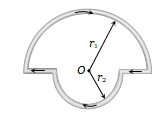In the figure shown there are two semicircles of radii ${\mathrm{r}}_{1}$ and ${\mathrm{r}}_{2}$ in which a current i is flowing. The magnetic induction at the centre O will be(a)  $\frac{{\mathrm{\mu }}_{0}\mathrm{i}}{\mathrm{r}}\left({\mathrm{r}}_{1}+{\mathrm{r}}_{2}\right)$                            (c)  $\frac{{\mathrm{\mu }}_{0}\mathrm{i}}{4}\left[\frac{{\mathrm{r}}_{1}+{\mathrm{r}}_{2}}{{\mathrm{r}}_{1}{\mathrm{r}}_{2}}\right]$

(b)  $\frac{{\mathrm{\mu }}_{0}\mathrm{i}}{4}\left({\mathrm{r}}_{1}-{\mathrm{r}}_{2}\right)$                             (d) $\frac{{\mathrm{\mu }}_{0}\mathrm{i}}{4}\left[\frac{{\mathrm{r}}_{2}-{\mathrm{r}}_{1}}{{\mathrm{r}}_{1}{\mathrm{r}}_{2}}\right]$